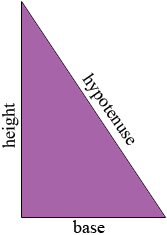SEARCH HOMEMath Central Quandaries & QueriesQuestion from Anah, a student: Could you please tell me the formula for me to calculate the height of a triangle. I have the angles and side lengths. I am trying to calculate the height of an right triangle, the dimensions are as follows: The Base/Hypotenuse is: 8x Side A is 2x. Side B is 2x.Anah,

If you rotate a right triangle so that the base is one of its legs then the height is the length of the other leg.The dimensions you sent however describe an impossible triangle. In any triangle the sum of the lengths of any two of the sides must be larger than the length of the third side. But 2x + 2x is not greater than 8x. Hence there is no triangle with side lengths 2x, 2x and 8x.

I hope this helps,
HarleyMath Central is supported by the University of Regina and The Pacific Institute for the Mathematical Sciences.